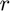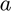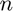# imod

Remainder after division with divisor's sign (complexified version of mod).

## Syntax

```r = imod(a,n)
```

## Description

r = imod(a,n) returns the remainder,, after division ofby(with's sign). Note thatcan be complex, but it is assumed thatis real.

## Input/Output Parameters

 Variable Symbol Description Format Units Input a dividend 1×1complex - n divisor 1×1double - Output r remainder of (with divisor's sign) 1×1complex -